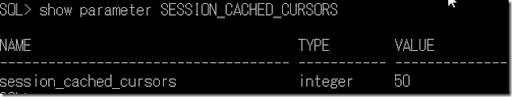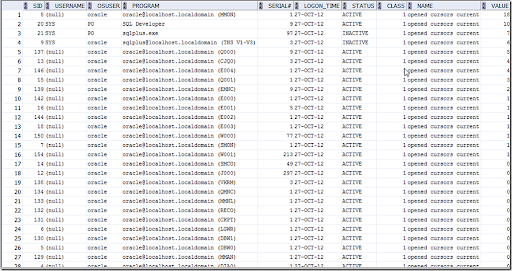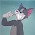## Sunday, October 28, 2012

### 3Cursor

Every query comes into oracle, oracle would assign the cursor(s) in PGA’s private SQL area. OPEN_CURSORS parameter define how many cursors can open by a user sessions.

More cursors open, used more memory in PGA.

#### SESSION_CACHED_CURSORS

Number of cursors a session can store. 11.2 default value is 50. This is to avoid the soft parse.#### Show cursors number by applications and users

` `
`    SELECT`
`      s.sid,`
`      s.username,`
`      s.osuser,`
`      S.program,`
`      S.SERIAL#,`
`      S.LOGON_TIME,`
`      S.STATUS,`
`      B.class,`
`      b.name,`
`      v.value`
`    from`
`      V\$SESSTAT v,`
`      v\$statname b,`
`      V\$SESSION S,`
`      v\$process p`
`    where`
`    v.statistic# = b.statistic#`
`    and s.paddr = p.addr`
`    and V.sid=S.sid`
`    -- and s.username is not null`
`    and B.name='opened cursors current'`
`     -- and value > 10 `
`    order by`
`     10 desc`#### Show the open cursor’s SQL

`SELECT`
`  s.sid,`
`  s.username,`
`  s.osuser,`
`  S.program,`
`  S.SERIAL#,`
`  S.LOGON_TIME,`
`  S.STATUS,`
`  B.class,`
`  b.name,`
`  V.value,`
`  sql.SQL_TEXT`
`FROM`
`  V\$SESSTAT v,`
`  v\$statname b,`
`  V\$SESSION S,`
`  V\$PROCESS P,`
`  V\$SQLTEXT SQL`
`WHERE`
`  sql.ADDRESS    = S.SQL_ADDRESS`
`AND v.statistic# = b.statistic#`
`AND s.paddr      = p.addr`
`AND V.sid        =S.sid`
`AND B.name       ='opened cursors current'`
`ORDER BY`
`  p.spid,`
`  sql.hash_value,`
`  sql.piece`1.2.3.